##### Trigonometry For Dummies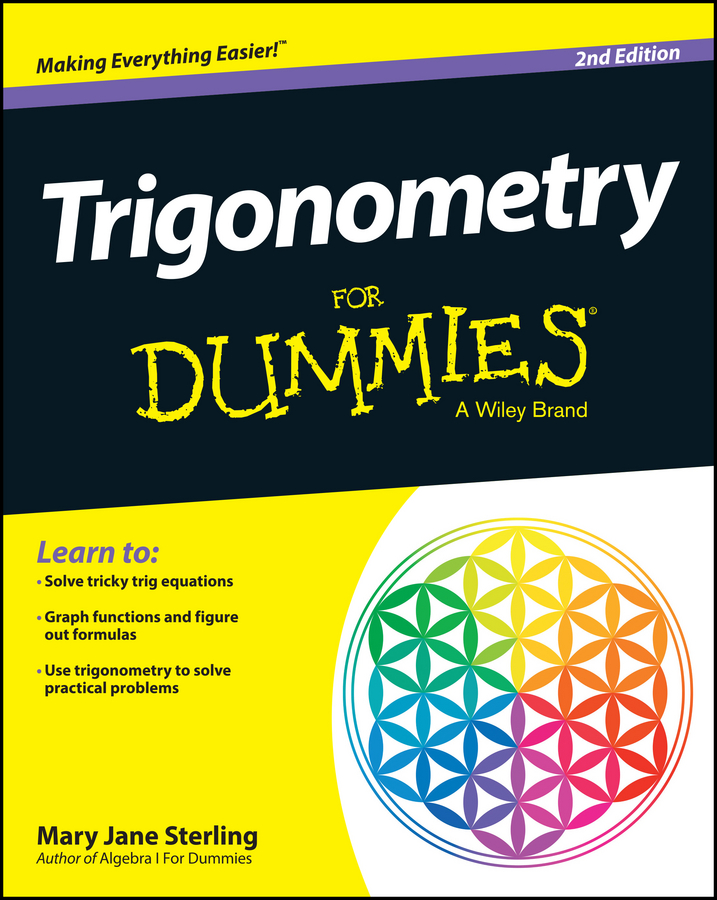You can identify similar polygons by comparing their corresponding angles and sides. As you see in the following figure, quadrilateral WXYZ is the same shape as quadrilateral ABCD, but it’s ten times larger (though not drawn to scale, of course). These quadrilaterals are therefore similar.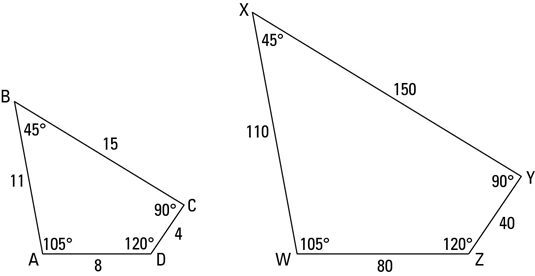Similar polygons: For two polygons to be similar, both of the following must be true:

• Corresponding angles are congruent.

• Corresponding sides are proportional.

To fully understand this definition, you have to know what corresponding angles and corresponding sides mean. (Maybe you’ve already figured this out by just looking at the figure.) Here’s the lowdown on corresponding. In the figure, if you expand ABCD to the same size as WXYZ and slide it to the right, it’d stack perfectly on top of WXYZ.

A would stack on W, B on X, C on Y, and D on Z. These vertices are thus corresponding.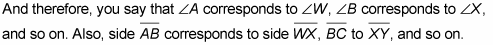In short, if one of two similar figures is expanded or shrunk to the size of the other, angles and sides that would stack on each other are called corresponding.

When you name similar polygons, pay attention to how the vertices pair up.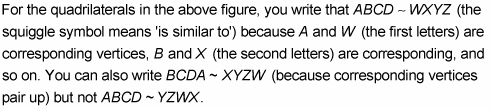Now use quadrilaterals ABCD and WXYZ to explore the definition of similar polygons in greater depth:

• Corresponding angles are congruent.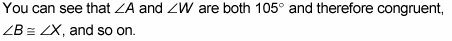When you blow up or shrink a figure (creating a figure similar to the original) the angles don’t change. Whether you’ve realized it or not, you’ve really known this since you were a little kid. Think about looking at a photo of something where the image in the photo is much smaller than the actual object (or imagine how things look bigger when you’re closer to them). If the angles in the photo were different than the angles in the original object, the photo wouldn’t look like the photographed object. It would look warped.

• Corresponding sides are proportional. The ratios of corresponding sides are equal, like this: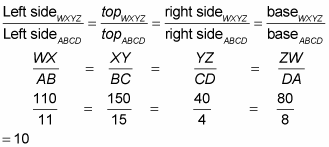Each ratio equals 10, the expansion factor. (If the ratios were flipped upside down — which is equally valid — each would equal 1/10, the shrink factor.) And not only do these ratios all equal 10, but the ratio of the perimeters of ABCD and WXYZ also equals 10.

Perimeters of similar polygons: The ratio of the perimeters of two similar polygons equals the ratio of any pair of their corresponding sides. (But note that the ratio of the areas of two similar polygons does not equal the ratio of corresponding sides.)